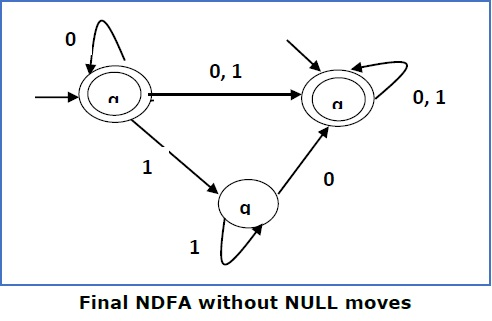# Construction of an FA from an RE

We can use Thompson's Construction to find out a Finite Automaton from a Regular Expression. We will reduce the regular expression into smallest regular expressions and converting these to NFA and finally to DFA.

Some basic RA expressions are the following −

Case 1 − For a regular expression ‘a’, we can construct the following FA −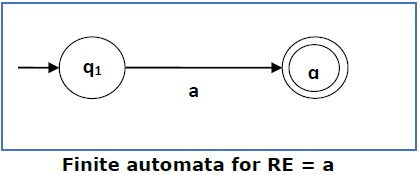Case 2 − For a regular expression ‘ab’, we can construct the following FA −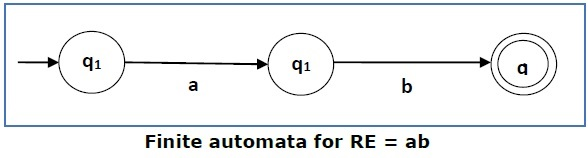Case 3 − For a regular expression (a+b), we can construct the following FA −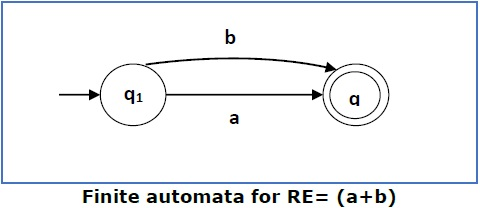Case 4 − For a regular expression (a+b)*, we can construct the following FA −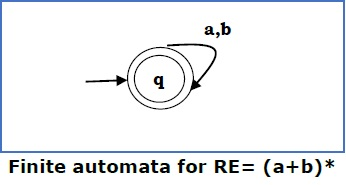### Method

Step 1 Construct an NFA with Null moves from the given regular expression.

Step 2 Remove Null transition from the NFA and convert it into its equivalent DFA.

Problem

Convert the following RA into its equivalent DFA − 1 (0 + 1)* 0

Solution

We will concatenate three expressions "1", "(0 + 1)*" and "0"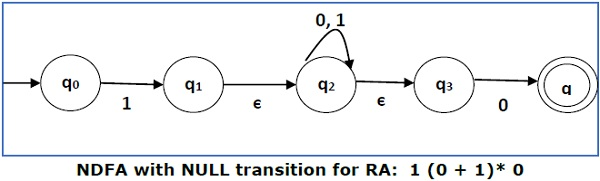Now we will remove the ε transitions. After we remove the ε transitions from the NDFA, we get the following −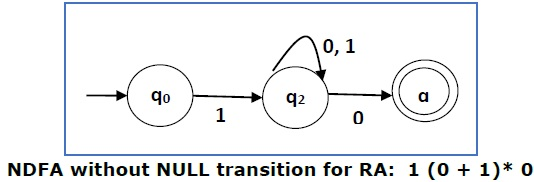It is an NDFA corresponding to the RE − 1 (0 + 1)* 0. If you want to convert it into a DFA, simply apply the method of converting NDFA to DFA discussed in Chapter 1.

## Finite Automata with Null Moves (NFA-ε)

A Finite Automaton with null moves (FA-ε) does transit not only after giving input from the alphabet set but also without any input symbol. This transition without input is called a null move.

An NFA-ε is represented formally by a 5-tuple (Q, ∑, δ, q0, F), consisting of

• Q − a finite set of states

• − a finite set of input symbols

• δ − a transition function δ : Q × (∑ ∪ {ε}) → 2Q

• q0 − an initial state q0 ∈ Q

• F − a set of final state/states of Q (F⊆Q).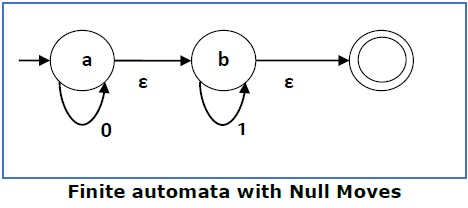The above (FA-ε) accepts a string set − {0, 1, 01}

## Removal of Null Moves from Finite Automata

If in an NDFA, there is ϵ-move between vertex X to vertex Y, we can remove it using the following steps −

• Find all the outgoing edges from Y.
• Copy all these edges starting from X without changing the edge labels.
• If X is an initial state, make Y also an initial state.
• If Y is a final state, make X also a final state.

Problem

Convert the following NFA-ε to NFA without Null move.Solution

Step 1

Here the ε transition is between q1 and q2, so let q1 is X and qf is Y.

Here the outgoing edges from qf is to qf for inputs 0 and 1.

Step 2

Now we will Copy all these edges from q1 without changing the edges from qf and get the following FA −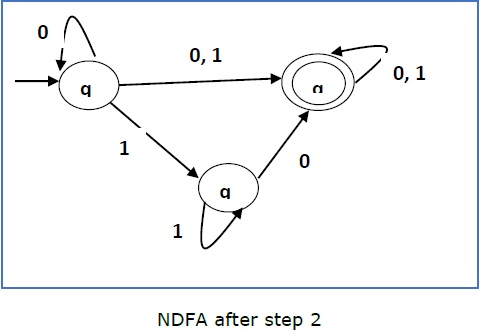Step 3

Here q1 is an initial state, so we make qf also an initial state.

So the FA becomes −Step 4

Here qf is a final state, so we make q1 also a final state.

So the FA becomes −